### Mathematica Lab Tutorials: Lab 11

### Lab Questions

Consider the following BVP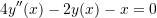With the boundary conditions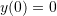and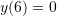• Find the exact solution using the built-in DSolve function in Mathematica.
• Use the finite difference method with n=5 intervals to find a numerical solution.
• Plot the exact solution overlapping the data points obtained using the numerical solution.
• Find the maximum absolute error.
• Write a procedure whose input isand the output is the maximum absolute error.
• Plot the curve of the maximum absolute error vs.for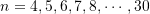• Plot the curve of the maximum absolute error vs.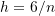for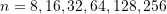• Fit the nonlinear model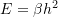to the data of maximum error vs.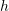(Note the error is expected to be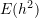because the basic formula for the centred finite difference scheme is used)

### Video Tutorials

#### Tutorial 1

Finding exact solutions using DSolve function. A review of FullSimplify and Solve functions. Introducing the Symbol function.

#### Tutorial 2

Using the finite difference method to solve differential equations.

#### Tutorial 3

An example of finding the error in the finite difference method as a function of the number of intervals.